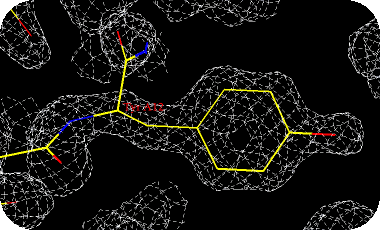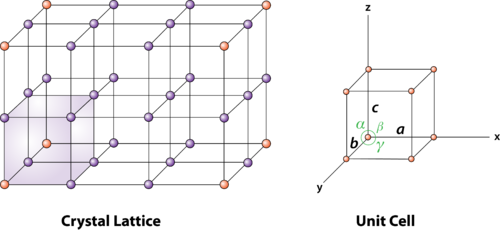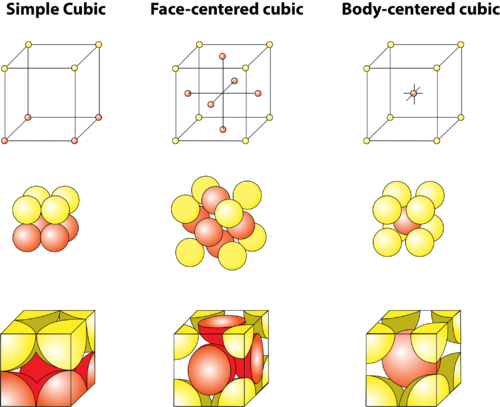Unit Cells

• Define unit cell.
• Describe the three cubic crystal system unit cells.How can we measure size of atoms?

We have many data tables available to us in chemistry. If we wanted to know the size of the lithium atom, we can easily look it up and find that this atom is 134 picometers across. If we remove the outer electron, the lithium ion is now only 90 picometers in size. How do we know this? We don’t have a ruler small enough to measure these tiny distances. But we can use a technique called x-ray diffraction to shine beams of x-rays through a crystal of a lithium compound. By measuring how much the beams are bent after they come through the crystal, we can calculate the size of the molecule. This technique works both for small materials like lithium compounds and equally well for very large protein molecules.

Unit Cells

A unit cell is the smallest portion of a crystal lattice that shows the three-dimensional pattern of the entire crystal. A crystal can be thought of as the same unit cell repeated over and over in three dimensions. The Figure below illustrates the relationship of a unit cell to the entire crystal lattice.Figure 13.16

A unit cell is the smallest repeating portion of a crystal lattice.

Unit cells occur in many different varieties. As one example, the cubic crystal system is composed of three different types of unit cells: (1) simple cubic , (2) face-centered cubic , and (3)body-centered cubic . These are shown in three different ways in the Figure below .Figure 13.17

Three unit cells of the cubic crystal system. Each sphere represents an atom or an ion. In the simple cubic system, the atoms or ions are at the corners of the unit cell only. In the face-centered unit cell, there are also atoms or ions in the center of each of the six faces of the unit cell. In the body-centered unit cell, there is one atom or ion in the center of the unit cell in addition to the corner atoms or ions.

Pay special attention to the last diagram for each type of cell. You will notice that the atoms or ions at the edges of each face or at the corners are not complete spheres. In the simple cubic cell, each corner atom is shared by 8 differenent unit cells. The same situation exists for the edge or corner particles in the face-centered and body-centered cubic forms. In addition, each of the particles in the center of the face-centered cubic cell is shared by 2 unit cells. Body-centered cells have an additional atom in the middle of the cell which is contained entirely in that cell.

Note that we have only considered the unit cells of a cubic crystal. Other crystal forms also have unit cells. These unit cells are listed below:

• rhombohedral, hexagonal, triclinic – one unique form each
• tetragonal – simple and body-centered
• monoclinic – simple and base-centered
• orthorhombic – simple, face-centered, body-centered, base-centered

Summary

• A unit cell is the smallest portion of a crystal lattice that shows the three-dimensional pattern of the entire crystal.
• There are three different types of unit cells in the cubic crystal system.

Practice

Questions

http://chemwiki.ucdavis.edu/Physical_Chemistry/Physical_Properties_of_Matter/Solids/Unit_Cell

1. How is a unit cell defined?
2. Opposite faces of a unit cell are __________.
3. What does the edge of a unit cell connect?

Review

Questions

1. What is a unit cell?
2. List the three cubic unit cells.
3. Does each unit cell exist as an entity by itself?
• body-centered cubic: There is one atom or ion in the center of the unit cell in addition to the corner atoms or ions.
• face-centered cubic: There are also atoms or ions in the center of each of the six faces of the unit cell.
• simple cubic: The atoms or ions are at the corners of the unit cell only.
• unit cell: The smallest portion of a crystal lattice that shows the three-dimensional pattern of the entire crystal.